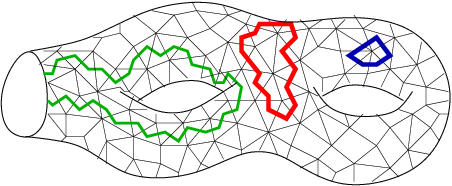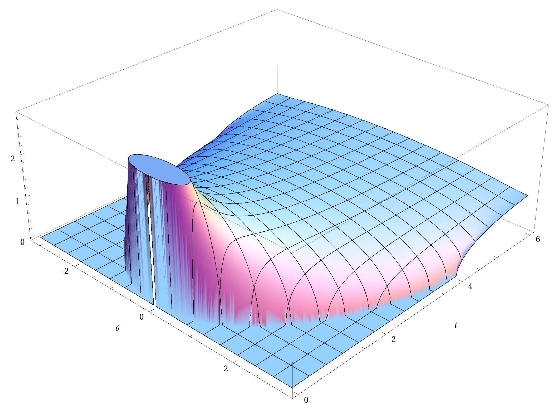# GranMa 2011

## Institut Henri Poincaré, Paris

General

Announcement

Participants

Practical details

Registration

Schedule

Leonid Chekhov, Steklov Math. Inst. Moscow and Concordia Univ. Montreal:

## External-field matrix model for Gaussian loop means

Using elementary resummations, we reduce the problem of finding muiltiloop means for Gaussian model to finding the partition function of the special one-matrix model with the external field of Kontsevich--Penner type. It is known that this model after the Miwa-type change of variables becomes equivalent to the one-matrix model with an arbitrary potential. What is lesser known is that, in terms of special times, it is related to reductions of moduli spaces and, in the same special times, it admits a decomposition into a pair of Kontsevich matrix models related by a canonical transformation. For the case of one loop mean (one backbone) we use the Harer-Zagier recursion formulas to (i) improve the recursion itself and (ii) solve it finding for example the first reduction coefficient for the moduli spaces of curves of arbitrary genus with one marked point.